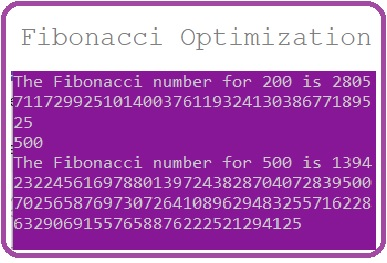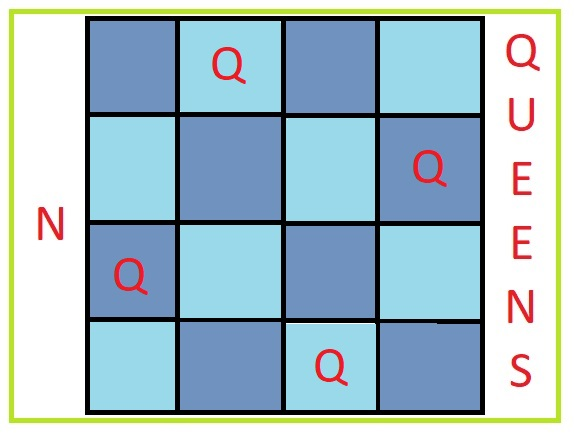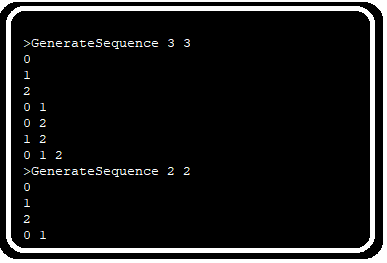# Fibonacci Series: Problem approach, fix Integer overflow and reduce time complexityIf you have ever done programming and went through some problems which need to be solved, you must have known the name of Fibonacci series. In the very first approach people just go through the problem statement which is:

“Fibonacci series is a series of numbers in which each number ( Fibonacci number ) is the sum of the two preceding numbers. The simplest is the series 1, 1, 2, 3, 5, 8, etc.”

In place of writing our own problem and finding the solutions let’s have a problem statement form the website OnlineJudge.org

https://onlinejudge.org/external/4/495.pdf

One can also submit the solution and test if it is acceptable or not at:

https://onlinejudge.org/index.php?option=com_onlinejudge&Itemid=8&page=submit_problem&problemid=436&category=0

We will not consider our solution complete until it gets accepted by the above link on sumbission.

As now a days, I am learning Java, I will prefer to code in java for this. I will try to use Java existing features so that, I don’t have to code specific difficult things. But I will go through the logic which might be required for this solution in C or C++ language without those features available.

## Coding the solution:

In formulae form we can say:

```F(n) = F(n-1) + F(n-2)
```

In this case finding the Nth term for the Fibonacci series looks easy as the statement is easy to understand. The naive approach of coding this function in Java would be:

```import java.io.*;
class Main{
static int fibonacci(int n){
int fib[]={0,1,1};
if(n<=2)
return fib[n];
return fibonacci(n-1)+fibonacci(n-2);
}
public static void main(String args[]) throws IOException{
String str="";
int number = Integer.parseInt(str);
System.out.println("The Fibonacci number for "+number+" is "+fibonacci(number));
}
}
}
```

So, the solution gives right output till some numbers and can be acceptable when you are a programmer who is new to programming. So, let’s submit this is online judge and check my status at website https://uhunt.onlinejudge.org/ I found that the solution gets error “Time Limit Exceeded”.

So, let’s see what happened. As per our problem we can have value of n till 5000 so for 5000 value we need to do all calculate of values below it. And if the problem set is having 5000 written multiple times our solution will calculate the value again and again. Just think, if I ask you the value of fibonacci(5000), 100 times will you do calculation again and again or you just write the value somewhere one time and then tell me the same value? Now for calculating fibonacci(5000), I also calculated fibonacci(4999), fibonacci(4998)… etc, so we can store all these values once we calculated it and then return it anytime when required. To sotre values let’s have an integer array which can store 5001 values, as we also want to store value of fibonacci(0). If this array has a non-zero value we will return the value from array else we will caculate it.

```import java.io.*;
class Main{
static Integer fib[]=new Integer;
static int fibonacci(int n){
if(n<=2)
return fib[n];
if(fib[n]==null){
fib[n]= fibonacci(n-1)+fibonacci(n-2);
}
return fib[n];
}
public static void main(String args[]) throws IOException{
fib=0;
fib=1;
fib=2;
String str="";
int number = Integer.parseInt(str);
System.out.println("The Fibonacci number for "+number+" is "+fibonacci(number));
}
}
}
```

Now when we submit above code in the OnlineJudge we don’t get “Time Limit Exceeded” error but we are getting wrong answer:

Let’s see some of the test cases, like random values 5000, 2900, 3999.. etc..

What we see is negative values. We all know that fibonacci series elements are all made of positive numbers so certainly this is a programming issue. As we are storing the values in integer variable it can’t only have values from -2147483648 to 2147483647. So any value greater than 2147483647 will be recorded as wrong value and will be converted into something integer can store. In this case we need data storage bigger than integer. So, let’s see Long which can store values from 0 to 264-1 which is 18446744073709551615. This is bigger than int but still for storing value of fibonacci(5000) we need a storage space to store 1000+ digits. This problem of coding is called integer overflow. Many modern languages like Python have inbuilt functionality to tackle integer overflow, so a python3 code will not give you such error. Luckily, Java now also have a utility BigInteger which can store long digit integers without overflow so let’s use BigInteger in our solution.

```import java.io.*;
import java.math.BigInteger;
class Main{
static BigInteger fib[]=new BigInteger;
static BigInteger fibonacci(int n){
if(n<=2)
return fib[n];
if(fib[n]==null){
}
return fib[n];
}
public static void main(String args[]) throws IOException{
fib=new BigInteger("0");
fib=new BigInteger("1");
fib=new BigInteger("1");
String str="";
int number = Integer.parseInt(str);
System.out.println("The Fibonacci number for "+number+" is "+fibonacci(number));
}
}
}
```

We got our solution accepted in this case. But if we are not working in Java and Python like C and C++ then we need to store the integer values in string and create a function to add those values and return new string. I have also worked on that code and make it available below:

```import java.io.BufferedReader;
import java.io.IOException;
class Main{
static String add(String num1, String num2){
String sum="";

long carry=0;
for(int i=num1.length(),j=num2.length();i>0 || j>0;i--,j--){
long digit1=0L,digit2=0L;
if(i!=0)
digit1=Long.parseLong(num1.substring(i-1,i));
if(j!=0)
digit2=Long.parseLong(num2.substring(j-1,j));
long intSum = digit1 + digit2 + carry;
sum=(intSum)%10L+sum;
carry=(intSum)/10L;
}
if(remainder>0){
sum=carry+sum;
}
return sum;
}
public static void main(String[] args) throws IOException{
String str="";
String[] fibonacci = new String;
fibonacci="0";
fibonacci="1";
for(int i=2;i<5001;i++){
}
while ((str = br.readLine()) != null)
{
int number = Integer.parseInt(str);
//number  = 10;
System.out.println("The Fibonacci number for "+number+" is "+fibonacci[number]);
}
}
}
```

This solution also get’s accepted into OnlineJudge.org website. This uses our primary class logic of adding digit by digit and store the values in string.

## Extra: Logic to improve string based storage for integers

If you look closely into the logic you can find that we have used strings are array of digits stored as characters. So, we are performing operation on every digit. In place of performing operation on every digit if we take an array of Long we can reduce number of operations in the process. This is an extra task and need not be required, so I will continue updating this area, as I am able to solve the problem this way or as it progresses.

Edit: I am able to develop the logic using Long array and the code worked faster than java BigInteger implementation, so the work has somehow succeeded, but still this is not the fastest solution available on OnlineJugde.org server.

```import java.io.BufferedReader;
import java.io.IOException;
class Main{
static final int maxPlaces=63;
static BigInteger fib[]=new BigInteger;
static class BigInteger{
long maxValToStoreInOneLong = 1000000000000000000L;
Long maxValToStoreInOneLongDivideByTenMinusOne = ((maxValToStoreInOneLong/10L)-1);
int maxLongDigits = maxValToStoreInOneLongDivideByTenMinusOne.toString().length();
Long[] arr = new Long[maxPlaces];

public BigInteger(Long val){
this.arr[maxPlaces-1]=val;
for(int i=maxPlaces-2;i>=0;i--){
this.arr[i]=0L;
}
}

BigInteger num1=this;
BigInteger sum = new BigInteger(0L);
int i=0;
long carry=0L;
for(i=maxPlaces-1; i>=0 ;i--){
long long1=0L, long2=0L;
if(num1.arr[i]==0L && num2.arr[i]==0L && carry==0L)
break;
long longSum = num1.arr[i] + num2.arr[i] + carry;
carry=0L;
if(longSum >= maxValToStoreInOneLong)
{
sum.arr[i]=longSum%maxValToStoreInOneLong;
carry = longSum/maxValToStoreInOneLong;
}else{
sum.arr[i]=longSum;
}
}
return sum;
}
public void printBigInt(){
for(int i=0;i<maxPlaces;i++){
System.out.print(this.arr[i]);
}
System.out.println();
}
public String toString(){
String number = "";
for(int i=maxPlaces-1;i>=0;i--){
if(this.arr[i]!=0L){
number=this.arr[i]+number;
if(this.arr[i-1]!=0L && this.arr[i] < (maxValToStoreInOneLong/10L)){
for( int j=maxLongDigits-(this.arr[i].toString().length());j>=0;j--){
number="0"+number;
}
}
}
}
if(number.length()==0)
return "0";
return number;
}
}
static BigInteger fibonacci(int n){
if(n<=2)
return fib[n];
if(fib[n]==null){
}
return fib[n];
}
public static void main(String args[]) throws IOException{
fib=new BigInteger(0L);
fib=new BigInteger(1L);
fib=new BigInteger(1L);
String str="";
int number = Integer.parseInt(str);
System.out.println("The Fibonacci number for "+number+" is "+fibonacci(number));
}
}
}
```

### Related Post## N Queens Problem – Iterative to recursive derivationN Queens Problem – Iterative to recursive derivation

There is a problem named N Queens which is famous in computer science courses and some programming challenges. Most of the websites who claim to explain the solution are either## Generate Combination SequenceGenerate Combination Sequence

Recently during a “Hiring Hackathon” of a company I went through a problem which required, to get all the combination of an array element. That is if the array length## Runtime Error in onlinejudge.org website for Java submissionRuntime Error in onlinejudge.org website for Java submission

I am using OnlineJudge.org website for years now but I used to submit my solutions in C++ and ANSI C. Recently I started submitting code in Python and there was